Question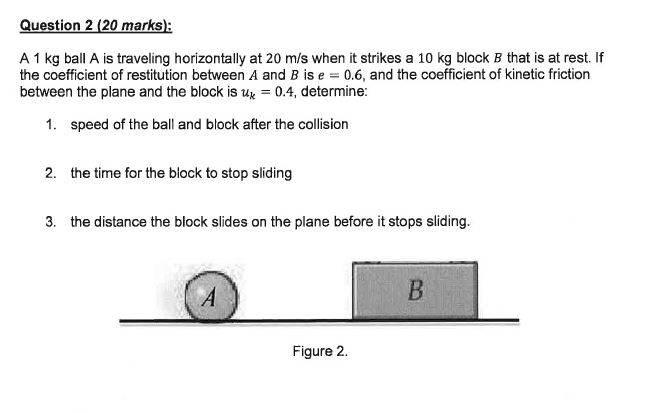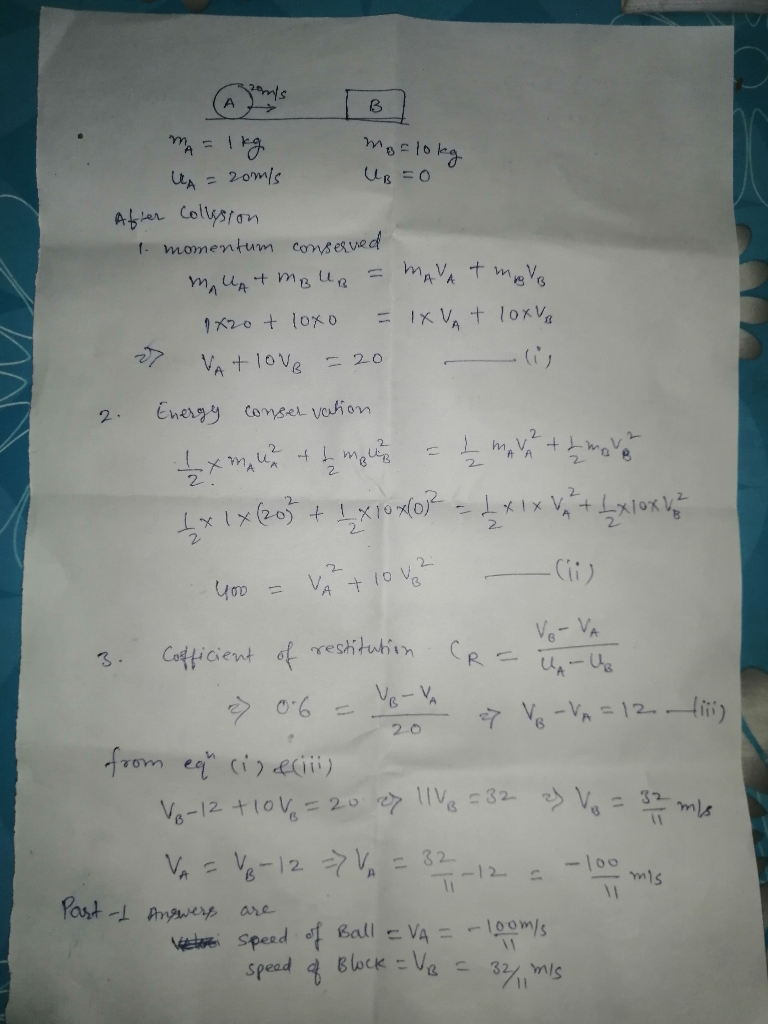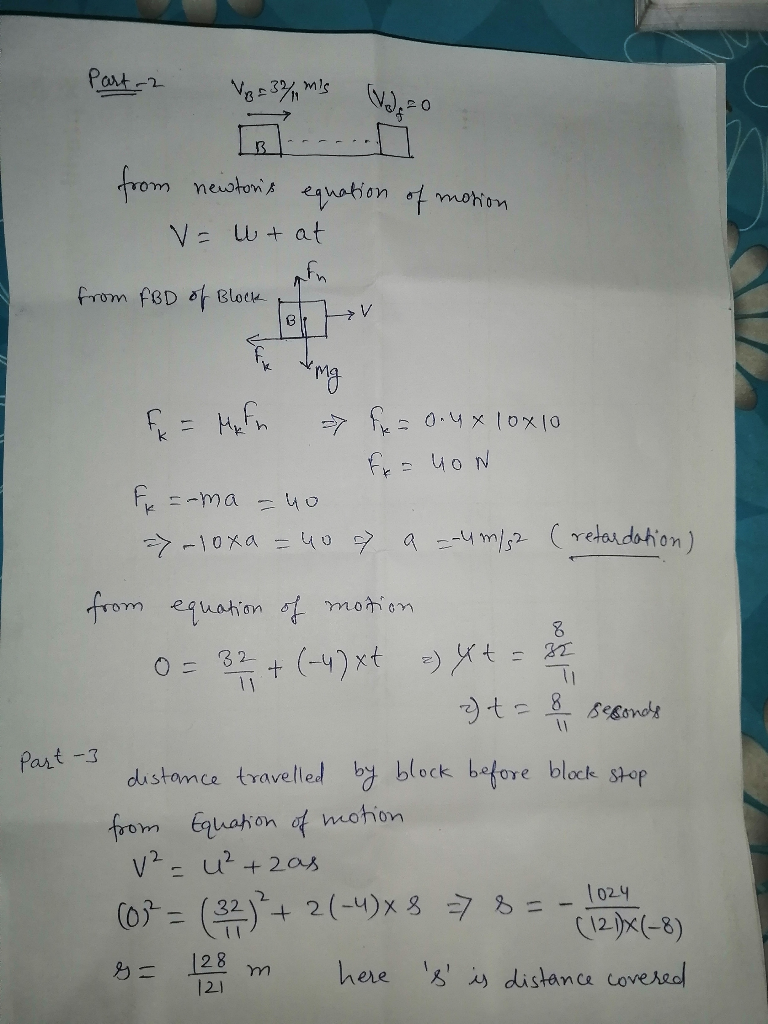Mainly concepts involved in this question are:

1. Conservation of momentum in collision

2. Conservation of Energy in Collision

3. Newton's equations of motion

#### Earn Coins

Coins can be redeemed for fabulous gifts.

Similar Homework Help Questions
• ### 7 of 7 A 3-lb ball A is traveling horizontally at 18 ft/s when it strikes...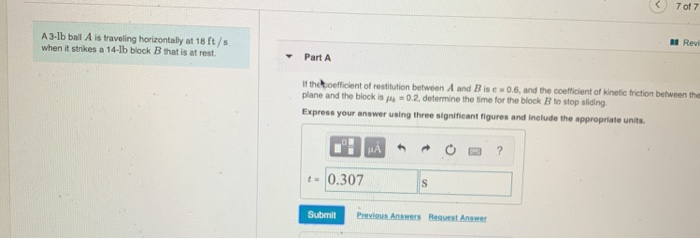7 of 7 A 3-lb ball A is traveling horizontally at 18 ft/s when it strikes a 14-lb block B that is at rest. Rev Part A If the efficient of restitution between A and B ise 0.6, and the coefficient of kinetic friction between the plane and the block is 0.2, determine the time for the block B to stop sliding Express your answer using three significant figures and include the appropriate units. H ? 10.307 S Submit Previous...

• ### A 15 gr bullet traveling at 500 m/s strikes the 5 kg wooden block and exists...

A 15 gr bullet traveling at 500 m/s strikes the 5 kg wooden block and exists the other side at 15 m/s as shown. The wooden block is initially at rest. Determine: a) The velocity of the wooden block just after the bullet exit it b) the average normal force on the wooden block if the bullets passes through it in 1.0 ms (millisecond) c) The time the block slides before it stops The coefficient of kinetic friction between the...

• ### The 20-g bullet is traveling at 400 m/s when it mes embedded in the 2-kg stationary...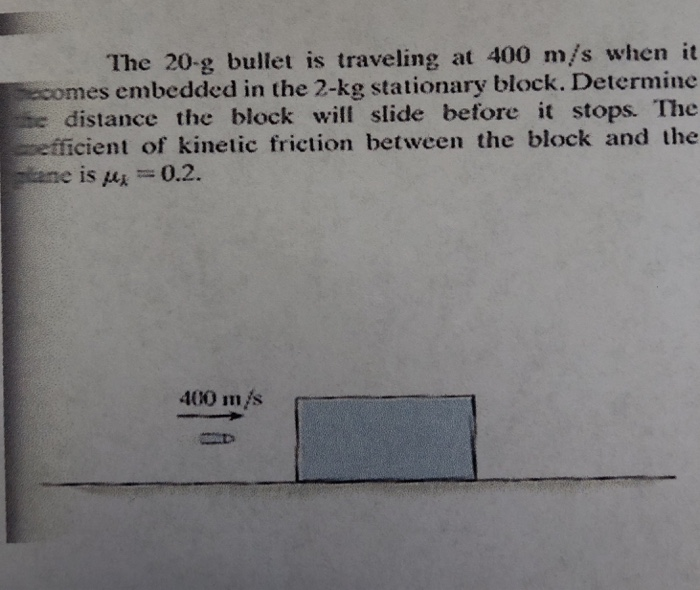The 20-g bullet is traveling at 400 m/s when it mes embedded in the 2-kg stationary block. Determine distance the block will slide before it stops. The pefficient of kinetic friction between the block and the ane is pu0.2. 400 m/s

• ### Question 3. A block A, having a mass of 20-kg, is released from rest and slides...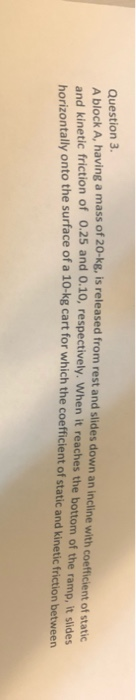Question 3. A block A, having a mass of 20-kg, is released from rest and slides down an incline with coeffici an incline with coefficient of static d kinetic friction of 0.25 and 0.10, respectively. When it reaches the bottom of the ramp, it slides ally onto the surface of a 10-kg cart for which the coefficient of static and kinetic friction between Question 3. A block A, having a mass of 20-kg, is released from rest and slides down...

• ### A 6.0 N force acts horizontally on a 20 N block that is initially at rest on a horizontal...

A 6.0 N force acts horizontally on a 20 N block that is initially at rest on a horizontal surface. The coefficient of static friction between the block and the surfaceis 0.6. The coefficient of kinetic friction between the block and the surface is 0.4. What is the magnitude of the frictional force that acts on the block?

• ### A steel ball of mass m = 1 kg and a cord of negligible mass and...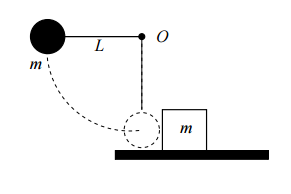A steel ball of mass m = 1 kg and a cord of negligible mass and length L = 2 m make up a simple pendulum that can pivot without friction about the point O (see below). This pendulum is released from rest in a horizontal position and when the ball is at its lowest point it strikes a block of mass m = 1 kg sitting at rest on a shelf. Assume that the collision is perfectly elastic and...

• ### A bullet of mass 0.01 kg is moving horizontally with a speed of 100 m/s when it hits a block of mass 2 kg that is at rest on a horizontal surface with a coefficient of friction of 0.4

A bullet of mass 0.01 kg is moving horizontally with a speed of 100 m/s when it hits a block of mass 2 kg that is at rest on a horizontal surface with a coefficient of friction of 0.4. After the collision the bullet becomes embedded in the block.

• ### A bullet of mass 4.3 g is fired horizontally into a 7.4 kg wooden block at...

A bullet of mass 4.3 g is fired horizontally into a 7.4 kg wooden block at rest on a horizontal surface. The coefficient of kinetic friction between block and surface is 0.53. The bullet stops in the block, which slides straight ahead for 1.7 m (without rotation). (a) What is the speed of the block immediately after the bullet stops relative to it? (b) At what speed is the bullet fired?

• ### A 0.0260 kg bullet moving horizontally at 450 m/s embeds itself into an initially stationary 0.500...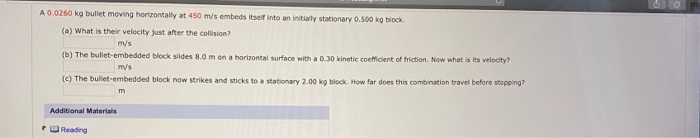A 0.0260 kg bullet moving horizontally at 450 m/s embeds itself into an initially stationary 0.500 kg block (a) What is their velocity just after the collision? m/s (b) The bullet-embedded block slides 8.0 m on a horizontal surface with a 0.30 kinetic coefficient of friction. Now what is its velocity? my's (c) The bullet embedded block now strikes and sticks to a stationary 2.00 kg block. How far does this combination travel before stopping? חח Additional Materials Reading

• ### A0.0240 kg bullet moving horizontally at 500 m/s embeds itself into an initially stationary 0.500 kg...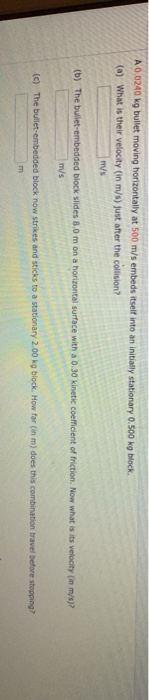A0.0240 kg bullet moving horizontally at 500 m/s embeds itself into an initially stationary 0.500 kg block (a) What is their velocity (in m/s) just after the collision? m/s (b) The bulet-embedded biock slides 8.0 m on a horizontal surface with a 0.30 kinetic coefficient of friction, Now what is its velocity (in m/s)? m/s (c) The bullet-embedded block now strikes and sticks to a stationary 2.00 kg block. How far (in m) does this combination travel before stopping? m